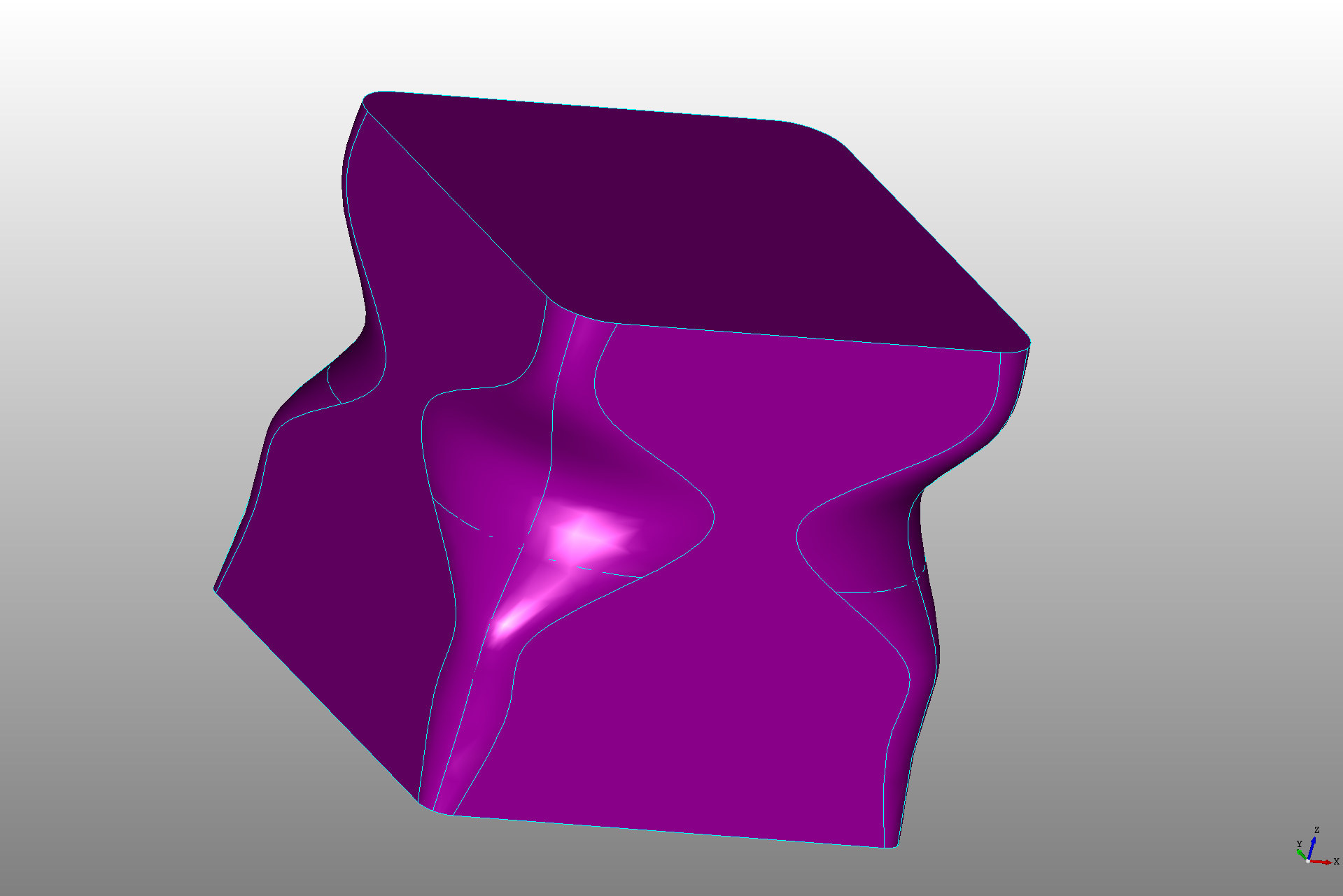## Fillet

The `Fillet` declaration can be used to add a radius to edges on a shape or vertices of a wire or face. There's several different types of fillet `operations` that can be performed so let's cover each.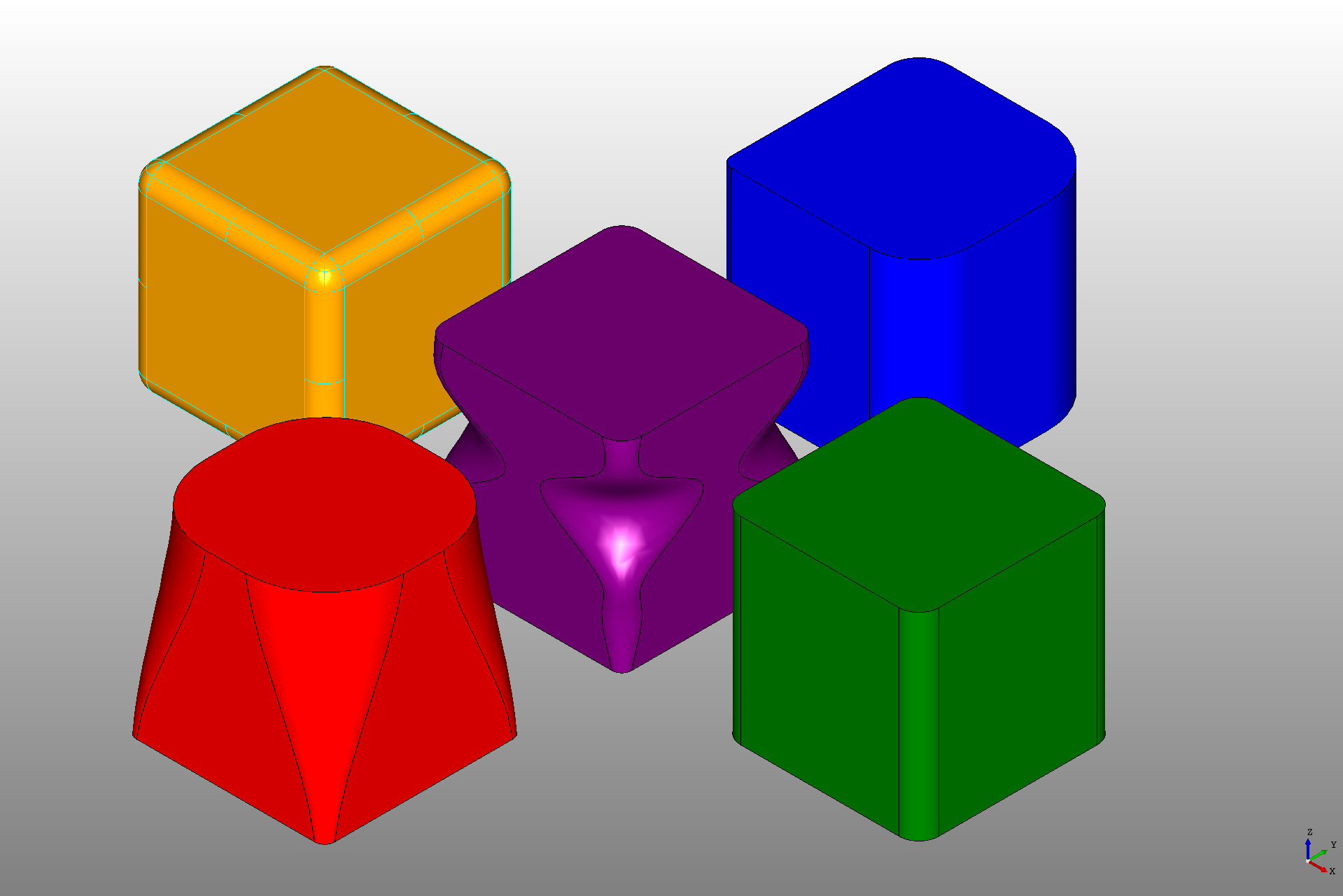The screenshot above demonstrates the following fillet operations:

• Filleting all edges of a shape with a fixed `radius` (shown in orange)
• Filleting only edges given in the `operations` list with the same `radius` attribute (shown in green)
• Filleting all edges of a face with a fixed `radius` (not shown above)
• Filleting specific edges with different radii by defining them in the `operations` list (shown in blue)
• Filleting specific edges with start and stop radii by defining them in the `operations` list (shown in red)
• Filleting a radius profile on an edge (shown in purple)

The `operations` can contain any combination of the above.

Note: Since defining fillet operations on a child shape requires inspection of the child shape, you can use the `disabled = True` attribute to quickly disable all fillets and access the child shape topology in the viewer.

#### All edges or vertices

If the `operations` list is not given, the `Fillet` declaration requires that the `radius` be set and will attempt to fillet every edge or vertex of the shape nested in the block with that radius.

For example this creates the orange or yellow cube in the picture above.

``````from declaracad.occ.api import Part, Box, Fillet

enamldef Assembly(Part):
Fillet:
# A fillet without specifying edges applies the fillet to all edges
color = 'orange'
Box:
dx = 200
dy = 200
dz = 200
``````

Note: If the radius is too large for any of the edges an error will be raised and the shape will not be rendered.

If the child shape is a planar face or closed wire it will perform a 2d fillet. To fillet an open wire please specify the vertices to fillet using the operations list.

#### Specific vertices, edges, or the edges of faces

The `operations` attribute can be set to a list of edges or a list of faces. In this case the radius set by the `radius` attribute on the fillet object. If the item is a face, all edges of the face will be fillted otherwise an single edge is expected. If the child is a planar face or wire it will perform a 2d fillet.

For example:

``````from declaracad.occ.api import Part, Box, Fillet

enamldef Assembly(Part):
Fillet:
color = 'green'
# The edges to fillet can be specified, using the topology of the shape
# at the moment there's not a good way pick which edges
operations = [e for i, e in enumerate(box.topology.edges) if i in (0, 2, 4, 6)]
Box: box:
position = (300, 0, 0)
dx = 200
dy = 200
dz = 200
``````

In this case each edge is given a radius of 20. The `edges` can be accessed using the `topology.edges` attribute of a declaration shape. These must then be filtered to select the correct edges.

To do all edges of a face simply specify the face.

``````        Fillet:
operations = [
box.topology.faces  # Top face
]
Box: box:
dx = 10
dy = 12
dz = 10
``````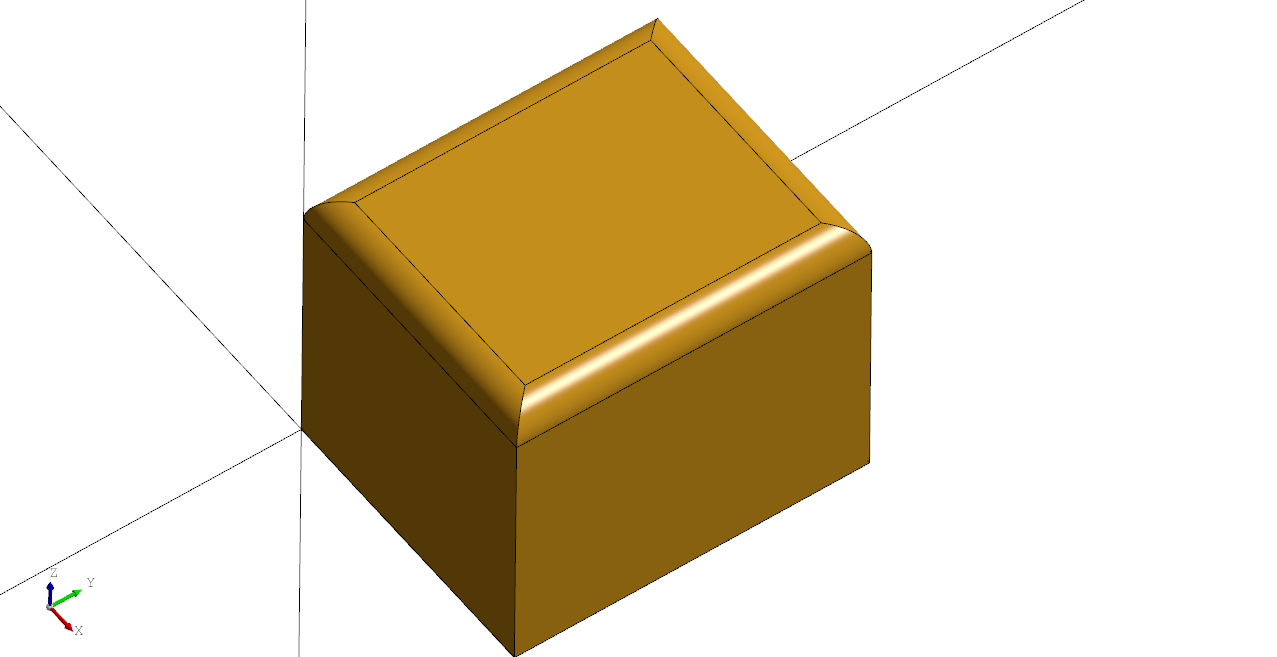Note: At the time of this writing there is not a good way to determine the proper edge (it is an area needing improvement). If the viewer selection mode is set to `edge` and you click the edge you want, the index of the edge will be printed in the output pane. Edges may also be filtered using the underlying OpenCASCADE handles.

This also works for vertices of a 2d plane or wire.

``````# Created in DeclaraCAD

enamldef Assembly(Part):
Fillet:
operations = rect.topology.vertices[0:2]
Rectangle: rect:
width = 10
height = 10
``````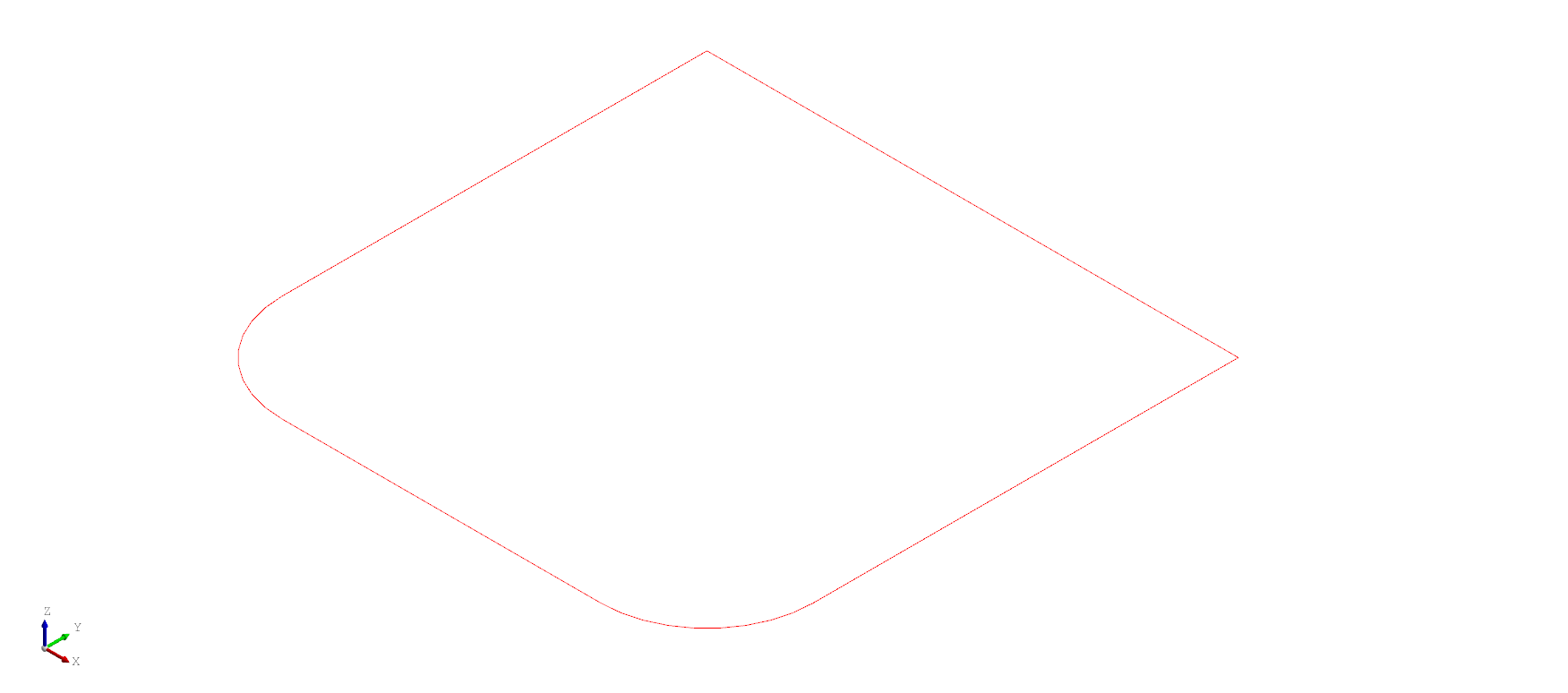#### Assigning a radius to a specific vertex, edge, or face

If an item in the list of `operations` is of the format `(radius, edge)`, it will fillet that edge with the specified radius. Likewise use the format `(radius, face)` to fillet all edges of a face and for planar faces or wires use `(radius, vertex)`.

For example:

``````from declaracad.occ.api import Part, Box, Fillet

enamldef Assembly(Part):
Fillet:
color = 'blue'
# You can also specify the radius for each edge by using a tuple
# for each edge in the format (radius, edge)
attr edges = box.topology.edges
operations = [
(10, edges),
(20, edges),
(30, edges),
(40, edges),
]
Box: box:
dx = 200
dy = 200
dz = 200
``````

Which fillets the radius of each edge as shown from the top view of the box below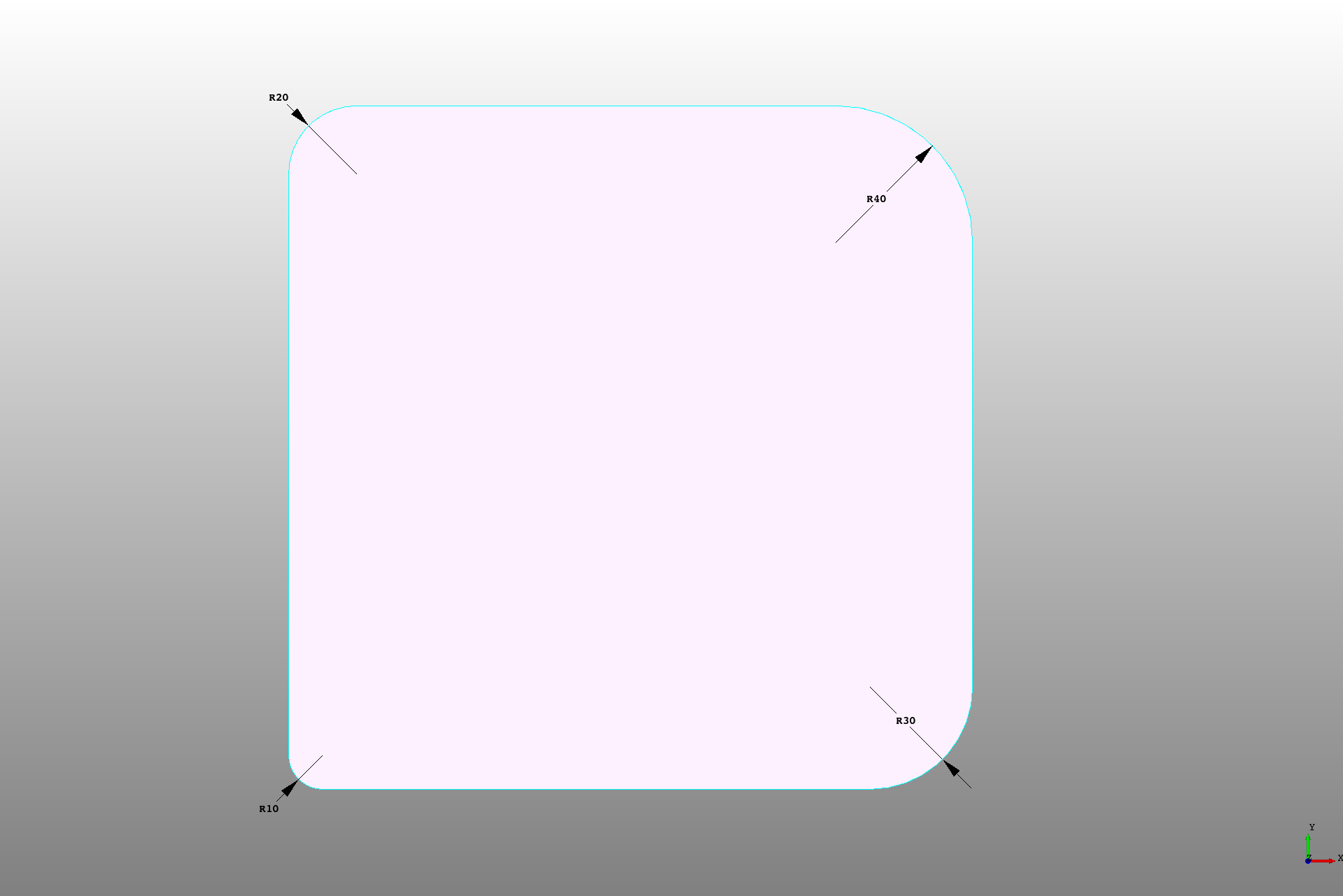Likewise for a planar face or wire.

``````# Created in DeclaraCAD

enamldef Assembly(Part):
Fillet:
operations = [
(1, rect.topology.vertices),
(4, rect.topology.vertices),
]
Face:
Rectangle: rect:
width = 10
height = 10
``````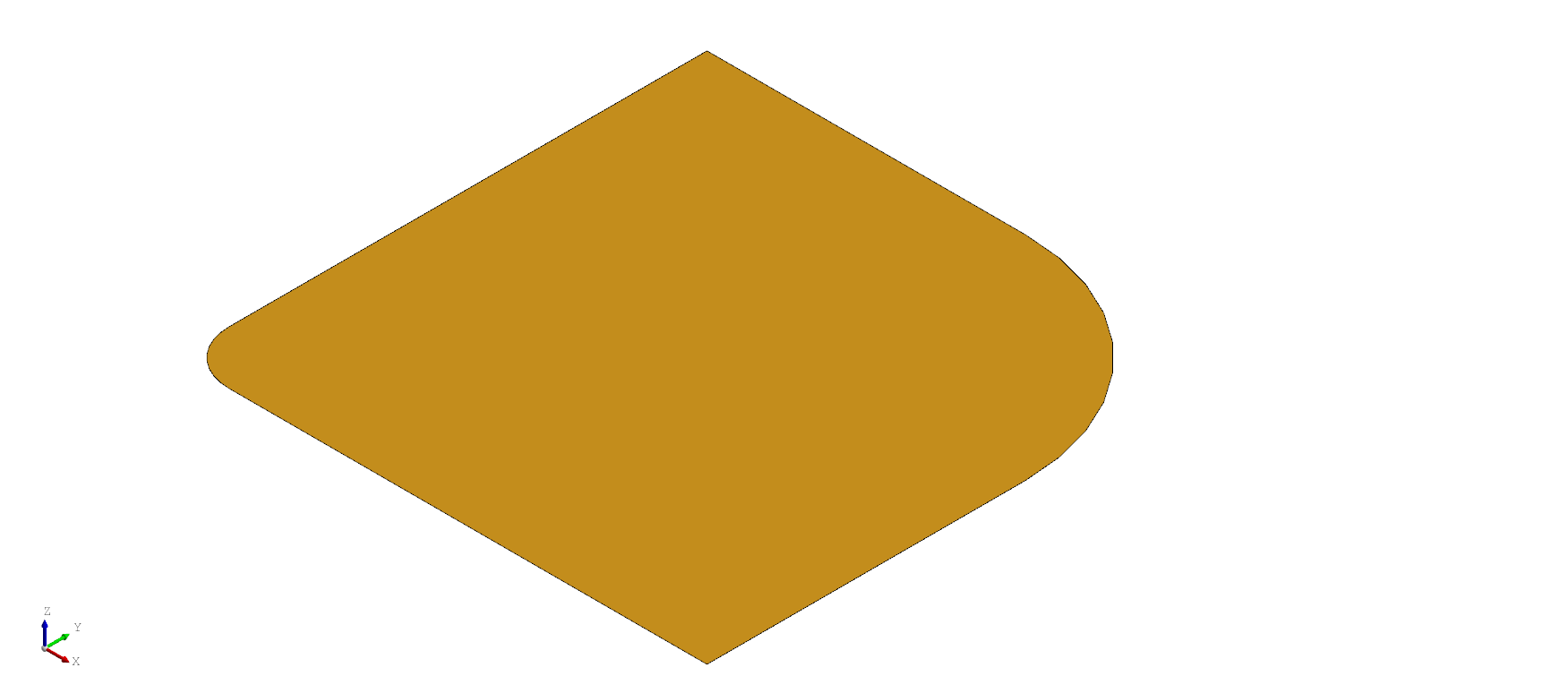#### Assigning a start and stop radius to a specific edge

If an item in the list of `operations` is of the format `(start_radius, stop_radius, edge)` it will apply a fillet to that edge with the specified start and stop radii.

``````from declaracad.occ.api import Part, Box, Fillet

enamldef Assembly(Part):
Fillet:
# You can also specify the start and end radius' for each edge
# by using tuples with the format (r1, r2, edge)
color = 'red'
attr r1 = 10
attr r2 = 80
operations = [(r1, r2, e)
for i, e in enumerate(box.topology.edges)
if i in (0, 2, 4, 6)]
Box: box:
position = (0, -300, 0)
dx = 200
dy = 200
dz = 200
``````

Which creates this: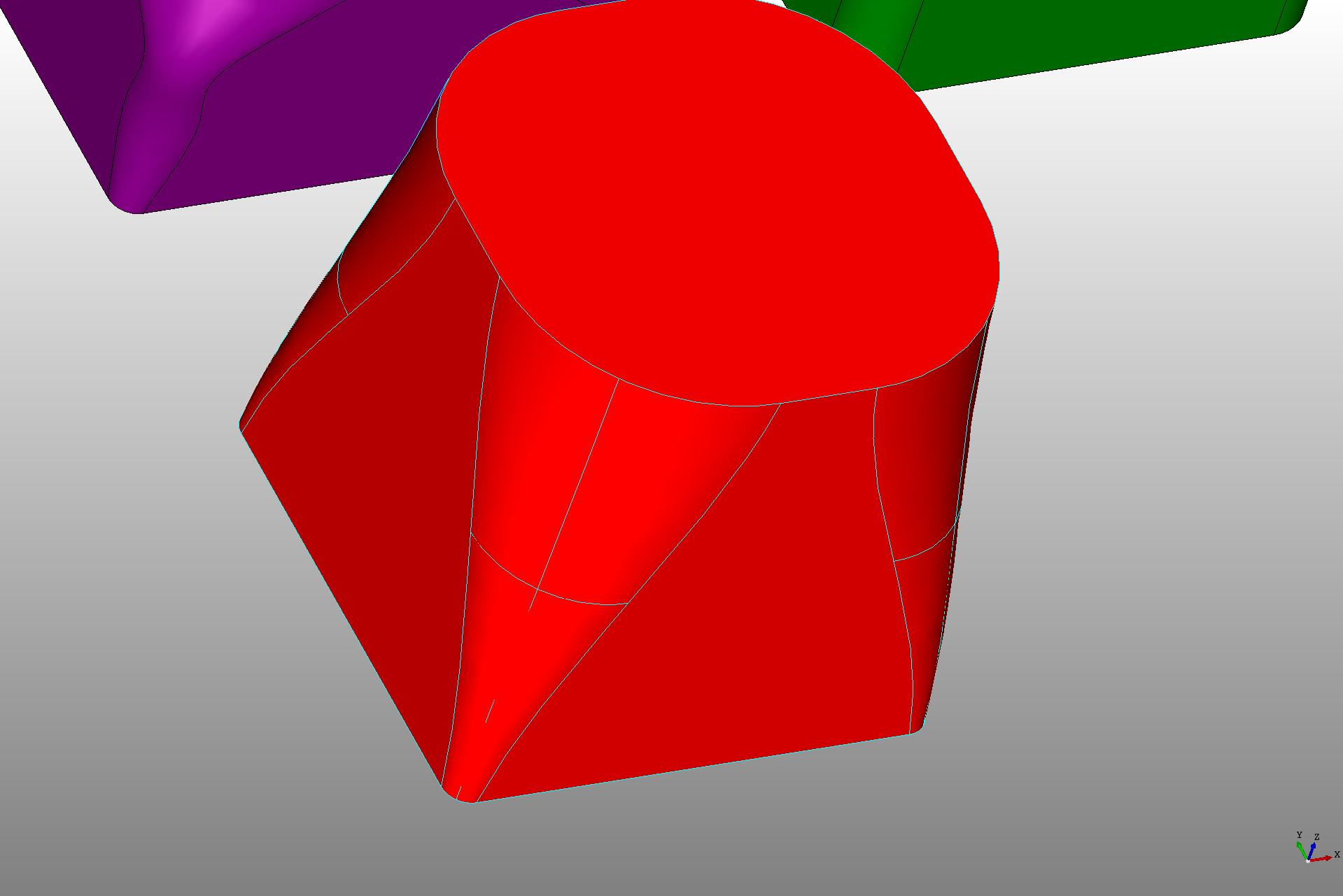#### Assigning a fillet profile to an edge

If an item in the list of `operations` is of the format `(profile, edge)` it will apply a fillet profile to that edge. In this case the `profile` needs to be a list of tuples of the format `(position, radius)` which define the fillet radius at the given position along the edge.

``````from declaracad.occ.api import Part, Box, Fillet

enamldef Assembly(Part):
Fillet:
# You can specify a set of points to fillet using a dynamic radius
color = 'purple'

# The profile is the position along the edge and the radius at that
# position
attr fillet_profile = [
(0, 10),
(50, 20),
(70, 20),
(130, 80),
(160, 30),
(200, 20),
]
operations = [(fillet_profile, e)
for i, e in enumerate(self.children.topology.edges)
if i in (0, 2, 4, 6)]
Box:
dx = 200
dy = 200
dz = 200
``````